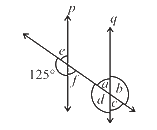# Ex.5.2 Q3 Lines and Angles - NCERT Maths Class 7

Go back to  'Ex.5.2'

## Question

In the adjoining figure, $$p || q$$. Find the unknown anglesVideo Solution
Lines & Angles
Ex 5.2 | Question 3

## Text Solution

Reasoning:

First, by using linear pair find the measure of $$\angle e$$ and then find the corresponding and vertically opposite angle to $$\angle e$$ and again by using linear pair find the value of $$\angle b$$ and its vertically opposite angle.

Steps:

Given $$p || q$$ and it is intersected by a transversal.

$$\angle = 125^\circ\\ \text{(Corresponding angle)}$$

Since,

$$125^\circ+ \angle e = 180^\circ \\ \text{(Linear pair)}$$

$$\angle e = 180^\circ - 125^\circ$$

$$\angle e = 55^\circ$$

$$\angle e = \angle f = 55^\circ \\ \text{(Vertically opposite angles)}$$

$$\angle e = \angle a = 55^\circ \\ \text{(Corresponding angles)}$$

$$\angle a + \angle b = 180^\circ \\ \text{ (Linear pair)}$$

$$55^\circ + \angle b = 180^\circ$$

$$\angle b = 180^\circ - 55^\circ$$

$$\angle b =125^\circ$$

Also,

$$\angle b = \angle d = 125^\circ \\ \text{(Vertically opposite angles)}$$

$$\angle a = \angle c = 55^\circ \\ \text{(Vertically opposite angles)}$$

Thus,

$$\angle a = 55^\circ; \angle b = 125^\circ; \angle c = 55^\circ;$$

$$\angle d = 125^\circ;\angle e = 55^\circ ; \angle f = 55^\circ$$

Learn from the best math teachers and top your exams

• Live one on one classroom and doubt clearing
• Practice worksheets in and after class for conceptual clarity
• Personalized curriculum to keep up with school